The OpenFOAM Foundation
complex Class Reference

Extension to the c++ complex library type. More...

Collaboration diagram for complex: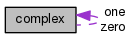[legend]

## Public Types

typedef complex cmptType
Component type. More...

## Public Member Functions

complex ()
Construct null. More...

complex (const scalar Re, const scalar Im)
Construct given real and imaginary parts. More...

complex (Istream &)
Construct from Istream. More...

scalar Re () const

scalar Im () const

scalar & Re ()

scalar & Im ()

complex conjugate () const

void operator= (const complex &)

void operator+= (const complex &)

void operator-= (const complex &)

void operator*= (const complex &)

void operator/= (const complex &)

void operator= (const scalar)

void operator+= (const scalar)

void operator-= (const scalar)

void operator*= (const scalar)

void operator/= (const scalar)

complex operator! () const

bool operator== (const complex &) const

bool operator!= (const complex &) const

## Static Public Attributes

static const char *const typeName = "complex"

static const complex zero

static const complex one

## Friends

scalar magSqr (const complex &c)

complex sqr (const complex &c)

scalar mag (const complex &c)

const complexmax (const complex &, const complex &)

const complexmin (const complex &, const complex &)

complex limit (const complex &, const complex &)

const complexsum (const complex &)

complex operator+ (const complex &, const complex &)

complex operator- (const complex &)

complex operator- (const complex &, const complex &)

complex operator* (const complex &, const complex &)

complex operator/ (const complex &, const complex &)

complex operator* (const scalar, const complex &)

complex operator* (const complex &, const scalar)

complex operator/ (const complex &, const scalar)

complex operator/ (const scalar, const complex &)

Istreamoperator>> (Istream &, complex &)

Ostreamoperator<< (Ostream &, const complex &)

## Detailed Description

Extension to the c++ complex library type.

Source files

Definition at line 76 of file complex.H.

## Member Typedef Documentation

 typedef complex cmptType

Component type.

Definition at line 86 of file complex.H.

## Constructor & Destructor Documentation

 complex ( )
inline

Construct null.

Definition at line 33 of file complexI.H.

Here is the caller graph for this function: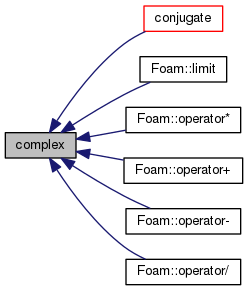complex ( const scalar Re, const scalar Im )
inline

Construct given real and imaginary parts.

Definition at line 37 of file complexI.H.

 complex ( Istream & is )

Construct from Istream.

Definition at line 39 of file complex.C.

## Member Function Documentation

 scalar Re ( ) const
inline

Definition at line 46 of file complexI.H.

Referenced by Foam::name().

Here is the caller graph for this function: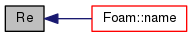scalar Im ( ) const
inline

Definition at line 52 of file complexI.H.

Referenced by Foam::name().

Here is the caller graph for this function: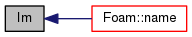scalar & Re ( )
inline

Definition at line 58 of file complexI.H.

 scalar & Im ( )
inline

Definition at line 64 of file complexI.H.

 complex conjugate ( ) const
inline

Definition at line 70 of file complexI.H.

References complex::complex().

Referenced by complex::operator!().

Here is the call graph for this function: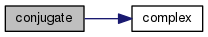Here is the caller graph for this function: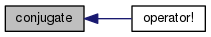void operator= ( const complex & c )
inline

Definition at line 78 of file complexI.H.

 void operator+= ( const complex & c )
inline

Definition at line 85 of file complexI.H.

 void operator-= ( const complex & c )
inline

Definition at line 92 of file complexI.H.

 void operator*= ( const complex & c )
inline

Definition at line 99 of file complexI.H.

References Foam::constant::universal::c.

 void operator/= ( const complex & c )
inline

Definition at line 105 of file complexI.H.

References Foam::constant::universal::c.

 void operator= ( const scalar s )
inline

Definition at line 111 of file complexI.H.

References s().

Here is the call graph for this function: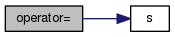void operator+= ( const scalar s )
inline

Definition at line 118 of file complexI.H.

References s().

Here is the call graph for this function: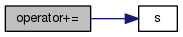void operator-= ( const scalar s )
inline

Definition at line 124 of file complexI.H.

References s().

Here is the call graph for this function: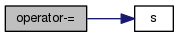void operator*= ( const scalar s )
inline

Definition at line 130 of file complexI.H.

References s().

Here is the call graph for this function: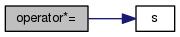void operator/= ( const scalar s )
inline

Definition at line 137 of file complexI.H.

References s().

Here is the call graph for this function: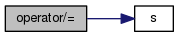complex operator! ( ) const
inline

Definition at line 144 of file complexI.H.

References complex::conjugate().

Here is the call graph for this function: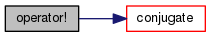bool operator== ( const complex & c ) const
inline

Definition at line 150 of file complexI.H.

References Foam::equal().

Referenced by complex::operator!=().

Here is the call graph for this function: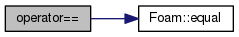Here is the caller graph for this function: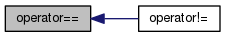bool operator!= ( const complex & c ) const
inline

Definition at line 156 of file complexI.H.

References complex::operator==().

Here is the call graph for this function: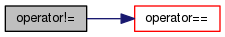## Friends And Related Function Documentation

 scalar magSqr ( const complex & c )
friend

Definition at line 165 of file complexI.H.

Referenced by Foam::mag(), and Foam::operator/().

 complex sqr ( const complex & c )
friend

Definition at line 171 of file complexI.H.

 scalar mag ( const complex & c )
friend

Definition at line 177 of file complexI.H.

Referenced by Foam::max(), and Foam::min().

 const complex& max ( const complex & c1, const complex & c2 )
friend

Definition at line 183 of file complexI.H.

 const complex& min ( const complex & c1, const complex & c2 )
friend

Definition at line 196 of file complexI.H.

 complex limit ( const complex & c1, const complex & c2 )
friend

Definition at line 209 of file complexI.H.

Referenced by Foam::limit().

 const complex& sum ( const complex & c )
friend

Definition at line 215 of file complexI.H.

 complex operator+ ( const complex & c1, const complex & c2 )
friend

Definition at line 232 of file complexI.H.

 complex operator- ( const complex & c )
friend

Definition at line 242 of file complexI.H.

 complex operator- ( const complex & c1, const complex & c2 )
friend

Definition at line 252 of file complexI.H.

 complex operator* ( const complex & c1, const complex & c2 )
friend

Definition at line 262 of file complexI.H.

 complex operator/ ( const complex & c1, const complex & c2 )
friend

Definition at line 272 of file complexI.H.

 complex operator* ( const scalar s, const complex & c )
friend

Definition at line 284 of file complexI.H.

 complex operator* ( const complex & c, const scalar s )
friend

Definition at line 290 of file complexI.H.

 complex operator/ ( const complex & c, const scalar s )
friend

Definition at line 296 of file complexI.H.

 complex operator/ ( const scalar s, const complex & c )
friend

Definition at line 302 of file complexI.H.

 Istream& operator>> ( Istream & , complex & )
friend
 Ostream& operator<< ( Ostream & , const complex & )
friend

## Member Data Documentation

 const char *const typeName = "complex"
static

Definition at line 91 of file complex.H.

 const Foam::complex zero
static

Definition at line 93 of file complex.H.

 const Foam::complex one
static

Definition at line 94 of file complex.H.

The documentation for this class was generated from the following files: